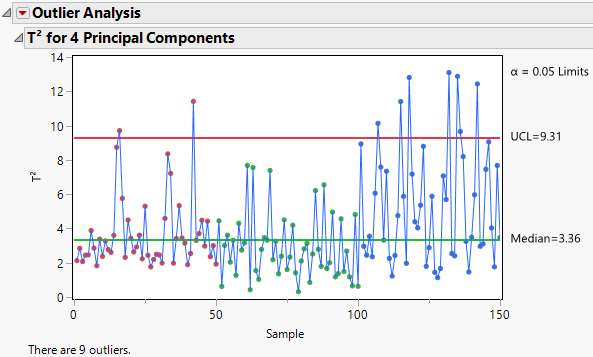Publication date: 11/10/2021

## Outlier Analysis

Figure 4.11 Outlier Analysis ReportBy default, the Outlier Analysis report displays the T2 for <A> Principal Components plot. The plot shows the T2 value for each observation and horizontal lines at the Median and Upper Control Limit (UCL). For more information about how the T2 values, median, and UCL are calculated, see Calculations for Outlier Analysis.

The α level used to calculate the UCL is displayed next to the plot. The number of outliers detected is shown below the plot. This number is the number of observations with T2 values that are greater than the UCL.

Tip: Hover over a point in the T2 Plot to view the T2 Contribution Proportion Plot for that observation. Click the T2 Contribution Proportion Plot to add it to the report window.

### Outlier Analysis Report Options

T2 Plot

Shows or hides the T2 plot. On by default.

Contribution Heat Map

Shows or hides a heat map of the T2 contribution values for all observations.

Contribution Proportions Heat Map

Shows or hides a heat map of the T2 contribution values for all observations expressed as a proportion of the individual row’s T2. The proportions for an individual row are obtained by computing the square of the contribution and dividing by the sum of the squares of all contributions for that individual row.

Contribution Plots for Selected Samples

(Available only if one or more points is selected in the T2 plot.) Shows a report with a T2 contribution plot for each selected sample. A T2 contribution plot shows the contribution of each variable to the sample’s T2 statistic. For more information about how the contributions are calculated, see Calculations for Outlier Analysis. Use contribution plots to investigate outliers. The variables with the largest positive or negative contributions are those that contribute most to a sample having a large T2 value. See Contribution Plots Report Options for information on red triangle menu options.

Contribution Proportion Plots for Selected Samples

(Available only if one or more points is selected in the T2 plot.) Shows a report with a T2 contribution proportion plot for each selected sample. A T2 contribution proportion plot shows the contribution values for the selected observations expressed as a proportion of the individual row’s T2. This is a different presentation of the information found in the Contribution Proportions Heat Map. See Contribution Plots Report Options for information on red triangle menu options.

Normalized DModX Plot

(Available only when the number of components is less than the number of variables.) Shows or hides a plot of the Normalized DModX values. DModX values are useful for detecting moderate outliers in the data.

Number of Components

Enables you to specify the number of principal components used in the T2 and T2 contribution statistics. When you change the number of components, the T2 plot, heap map, and normalized DModX plot automatically update.

Set α level

Enables you to specify the α level.

Save T2

Saves the T2 values to a new column in the data table.

Save Contributions

Saves the T2 Contributions to new columns in the data table. There is one column for each Y variable.

Save Normalized DModX

(Available only when the number of components is less than the number of variables.) Saves the normalized DModX values to a new column in the data table.

### Contribution Plots Report Options

The T2 Contribution Plots for Selected Samples, T2 Contribution Proportion Plots for Selected Samples, and T2 Mean Contribution Proportion Plots for Selected Samples contain the following red triangle menu options.

Bar Label

Displays a submenu of options to label the bars in the contribution plot. The label options are No Label, Label by Value, and Label by Column.

Remove Plot

Removes the contribution plot from the report.

Control Charts for Selected Items

(Available only if one or more bar segments are selected in the contribution plot.) Opens a Control Chart Builder window, with control chart results for each of the selected processes and groups.

Tip: You can also view the control chart by hovering over a bar segment in a contribution plot. Click the control chart to open the Control Chart Builder window.